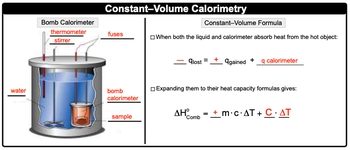Start typing, then use the up and down arrows to select an option from the list.## General Chemistry

Learn the toughest concepts covered in Chemistry with step-by-step video tutorials and practice problems by world-class tutors

8. Thermochemistry

# Constant-Volume Calorimetry

Constant-Volume Calorimetry uses a bomb calorimeter and a combustion reaction to determine its enthalpy of reaction.

Constant-Volume Calorimetry
1
concept

## Constant-Volume Calorimetry50s
Play a video:
here, we're going to say that the heat of combustion is the amount of heat released when a mole of a substance is burned or combusted. Now recall the combustion reaction normally involves a compound with carbon and hydrogen or carbon, hydrogen and oxygen reacting with 02 Of course, there are other elements that could be involved such a sulfur or nitrogen. But the most common types of combustion reactions are just carbon and hydrogen or carbon, hydrogen and oxygen. Now we're gonna say associated with any combustion reaction is a heat of combustion value, which we represent as Delta H Not so that little circle combustion so well, abbreviate it as comb. So just remember, when discussing constant value volume telemetry, we associated with a combustion reaction.

Heat of Combustion is the amount of heat released when 1 mole of substance is burned or combusted in a Bomb Calorimeter.

2
example

## Constant-Volume Calorimetry Example 159s
Play a video:
here, it says. Which of the following statements is true about the combustion off propane? So propane a C three h eight gas. It reacts with five moles of oxygen gas to produce three moles of carbon dioxide gas, plus four moles of water vapor. It has an entropy off combustion that's equal to negative 2222 killer jewels. Here, it says. It is Endo thermic. It is exile. Thermic. It absorbs heat from the surroundings or none of the above. So remember, a combustion reaction is accompanied with a negative Delta H value, which signifies that it is an ex. A thermic process. It releases heat to the surroundings. So it is X, a thermic not in doubt, thermic. And here it's releasing heat to the surroundings. If you're absorbing heat from the surroundings, you are not eggs. A thermic you are Indo thermic. So, out of all the choices on Lee, Option B is correct.
3
concept

## Constant-Volume Calorimetry2m
Play a video:
here, we're going to talk about a bomb cal or emitter. Now, a bomb calorie meter is a steel container with the combustible sample submerged in a known quantity of water. And we're going to say with a bomb cal or emitter, we have the concept of constant volume kalorama tree and this is used to determine the heat released during a combustion reaction. Now here, with this whole idea, we also have constant volume itself. This just means that the calorie meter has a fixed volume that doesn't expand after the sample is combusted. So just think of a combustion reaction as kind of a controlled explosion. So there's a explosion that goes on within the bomb calorie meter itself, but the volume stays fixed, It does not expand outward. And we're going to say, since it's a combustion reaction, that would mean that it's X A thermic. This would mean that our entropy of standard combustion for this reaction would be equal to negative Q. Lost, so negative Q. The amount of heat that's lost by the reaction. Now, if we take a look here, we're gonna first look at the bomb calorie meter itself, its components. So, with the bomb calorie meter, we're gonna have a top these two wires here. These are actually the fuses that helped to ignite the combustion reaction, we're gonna say here that they go down here and inside of this orange box, this represents our combustible sample. So we'll just say that this is our sample. This reaction occurs at some designated temperature and we know that through the use of a thermometer. So here are thermometers saying It's happening at 50°C here, we're going to say that this blue outline here is actually our water and the heat that's combusted that's released by our sample. We wanna make sure that heat is evenly distributed throughout the water sample. So we use this stir and then finally itself we have our our bomb calorie emitter. So our bank will remember, the sample is combusted, gives off heat, the heat exits the bank altimeter and it goes into the water, the water there, its temperature is read by the thermometer, the heat is dissipated evenly throughout the use of the stir. Now here with the bomb calorie meter, we also have our constant volume formula here, we're going to say this is when both the liquid and calorie meter absorb heat from the hot object here, we're going to say that it's equal to negative Q lost. So the amount of heat lost equals the amount of heat gained. So, gaining heat means Q. Is positive plus the calorie meter itself. Remember up here we said that and standard of combustion is equal to negative Q. Lost. So here these are the same. So negative Q. Lost equals our standard entropy of combustion. We're going to say that this amount of heat gained is related to this portion, since the heat gain is positive, that this m cat is also positive. And then we're going to finally say that our calorie meter itself, it uses our heat capacity, which is capital C. Times the change in temperature. So we would say that this portion, when we look at it, is the constant volume formula where our standard entropy of combustion equals positive, plus our heat capacity times change in temperature.4
example

## Constant-Volume Calorimetry Example 23m
Play a video: